E-Archive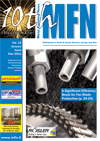Science Update

##### The Taguchi Methodology Used to Determine the Factor and Interaction Effects of the Controlled Shot Peening Process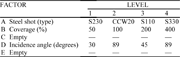Table 1: Summary of shot peen factors evaluated by design of experiments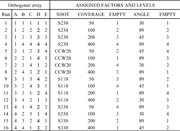Table 2: Fractional factorial array L16(45) with factors and levels assigned to columns. The orthogonal array was chosen from ref. .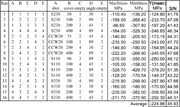Table 3: Results of the design of experiments analysis for the compressive residual stresses using the larger the better statistic as the MSD which measures the dispersion of the dataTable 4: Response table for residual stresses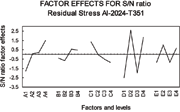Figure 1: S/N ratio for each effect level of the compressive residual stresses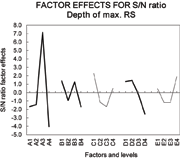Figure 2: S/N ratio for each effect and its level on depth of the maximum compressive residual stresses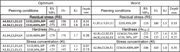Table 5: Estimated optimum and worst cases by using the DoE concepts

Abstract: A general methodology suitable for the optimisation of the different shot peening conditions that would enable a rational choice of the peening parameters has been used. The shot peening variables and their preferred levels can effectively be determined on a more scientific basis by means of an analysis of the effects induced on a particular component and the analysis of the interaction among these factors, rather than trusting in empirical considerations and experience.

1. Introduction

Design of experiments is an efficient experimental approach to studying the interaction effects between two or more factors in an experimental program also known as factorial design [1, 2]. Although design of experiments can take many forms, this strategy aims to quantitatively identify both the main effects of the factors and any interaction between them. The Classic and Taguchi methodologies have already been applied to address the choice of the parameters affecting the surface treatments, such as surface rolling and shot peening .

The effect of parameters set at various levels can be studied by following one of the better known experimental design approaches: (a) one factor at a time, (b) full factorial, (c) fractional factorial (orthogonal array experiments). Whereas all three may be used for studying the effects of the variables on the response of the system, not all are equally efficient. One factor at a time has been the traditional method used in academia and industry. However, it has been the most inefficient of all methods as it cannot evaluate any interaction among factors, it is not balanced and very frequently conclusions about factors are never reached and therefore, the optimisation process goes into a loop that does not always converge. Full factorial will require investigation of all possible combinations, and conclusions are usually apparent well before the experimental data are all collected. This approach is time-consuming and therefore, expensive. The fractional factorial (also called orthogonal array) is a method of setting up experiments that only requires a fraction of the full factorial combinations . It allows information to be obtained on all main effects and interactions, while keeping the size of the experiment manageable and also conducting it in a single, systematic effort. To bring this technique down to the ground level applications, the present study focuses on shot peening of engineering materials using an air blast shot peening machine. As peening intensity increases with increase in exposure, shot size and the incidence angle, then there should inherently be an effect on the response during individual variation of the process parameters. This work undertakes the study of the effect on peening effects such as stress concentration, compressive residual stresses and strain hardening when the abovementioned process parameters are varied simultaneously. Hence, aluminium alloy (AA) 2024-T351 was selected as work material in the present study since it is regularly used in automobile and aircraft industries.

2. Experiment design

A total of three factors were evaluated at four levels each as shown in table 1. A complete full factorial design that would allow for estimation of all main effects and factor interactions would require 43 or 64 experiments. Assuming that higher order interactions between factors were negligible, one-fourth fractional factorial design evolving a total of 16 experiments was selected.

A fractional factorial L16(45) array was used to enable the optimisation of five parameters with each of these set at a maximum of four levels. This approach permitted the estimation of all the main and first order factor interactions. The empty columns, third and fifth factors, were used to verify that all important parameters influencing shot peening effects were considered. The full fractional factorial array together with the selected factors and levels is depicted in table 2.

The evaluated effects are those obtained from: the measured-calculated residual stresses/depth (the hole drilling method), the direct measurement of the microhardness/depth and the calculated stress concentration factors (from the direct roughness measurements). The performance statistics utilised, i.e. the parameter MSD (mean square deviation), were selected according to the ensuing two modalities: (1) larger-the-better statistic for the maximum compressive residual stresses/depth and also for the work hardening (microhardness). (2) smaller-the-better statistic for the stress concentration (roughness).

3. Results and conclusions

Results of the analysis for the compressive residual stress in AA 2024-T351 are shown in table 3.

The S/N or signal to noise ratio (S/N) was calculated using “formula cannot be displayed online”

The best signal-to-noise ratio is that shown by the highest value, which in this case is run 11. It may be noted from the Y and S/N columns of table 3 that, although there is an overall agreement between Y and S/N, this agreement is not always held. This is because S/N includes dispersion, while Y does not. For this reason, the principal stresses (maximum and minimum) were used rather than solely Y.

In order to find the best combination, it was necessary to construct a response table, shown in table 4. This table includes Y and S/N from which are calculated Y' and S/N', required to determine the effect of the signal-to-noise (significant variation) of each factor for the whole experiment. Here, Y' and S/N' are the average of Ys and S/Ns for each factor level. For instance, the factor Y' for A1 (factor A, level 1) is computed as follows:
Y'A1 = (-124.8-233.7-197.2-246.65)/4 = -200.6, i.e. the average of all Ys for factor A at level 1 as illustrated by the shadowed values on the top left corner of table 4. SN' for factor A and level 1 is calculated in the same form.

The effect S/N for each level in the whole experiment is obtained from the difference between the averaged S/N (table 3) of the whole experiment and S/N' (table 4) for each factor and its corresponding level. Differently expressed, effect S/N' = S/N'-S/N. Thus, the effect of S/N' for the A1 = 44.15 - 45.93 = -1.8. The method of computing the effect of Y' for each factor at each level is analogous to that as for the effect S/N'.

The resulting incidence effect of S/N ratio for each factor regarding the compressive residual stresses is illustrated in Fig. 1. The control factor plot shows, in the first instance, the significant factors as those that present the highest variability of the calculated S/N' ratio. It is, therefore, observed that factor D (incidence angle) has the highest S/N' variation followed by factor A (shot). This means that incidence angle and shot type were the dominant factors for achieving the maximum compressive residual stresses. In order to evaluate the trustworthiness of this result, an analysis of variance approach was applied. The percentage contribution of the source to total variation determined for each of the factors was, for factor A (17%), B (3.5%), C (8.5%), D (62%) and E (9%).

The plots in Fig. 1 can also be used to estimate the optimum combination of factors and their corresponding levels to attain the predicted maximum residual stress; this is achieved by selecting the set points with the highest S/N' value. In particular, the combination A4, B3, C1, D2 and E2 (S330, 200% coverage and 89º incidence angle, according to table 1), would yield the maximum theoretical residual stress. This maximum residual stress is calculated by utilising the predictive model ( ypredicted = yexp + (yA - yexp) + (yB - yexp) + (yc - yexp) + ...) and the response table 4, as follows:
S/N' optimum = S/N average + Effects (SN')A maximum + Effects (SN')B maximum + Effects (SN')C maximum + Effects (SN')D maximum + Effects (SN')E maximum
N.B. All these values are shadowed in the response table 4.
Substitution of the numerical values into the predictive model, gives:
S/N' optimum = 45.93 + 1.5 + 0.6 + 1.3 + 2.6 + 1.0 = 52.93
Similarly, Y'optimum is calculated utilising the maximum compressive Effects Y' for each factor and the general average of Y, i.e.
Y'optimum = -224.86 – 32.3 – 37.6 – 35.0 – 54.2 – 17.9 = -401.86 MPa
Evidently, the above results are higher than the maximum experimental compressive residual stress found to be S/N = 51.35 and Y = -379.20 MPa (in run 11). The worst combination of peening conditions are obtained by considering the Effect S/N' or Effects Y' with the lowest values.
Following the same argument, shot size (factor A) is a significant factor when considering the depth to the maximum compressive residual stresses as depicted in Fig. 2.

Once the calculated optimum peening conditions are known for a particular peening effect, all the remainder peening effects either optimum or worst cases can be estimated by making use of the predictive model and the respective response tables for each of the remainder peening effects. For example, using the conditions A4, B3, C1, D2 and E2 (as determined above), the estimated values are: optimum residual stress = -402 MPa (as determined above); depth of the optimum RS = 0.31 mm; hardness = 150.5 Hv0.1 and stress concentration = 1.54. A summary of the estimated optimum and worst cases is given in table 5. In this table, the estimated peening conditions are categorised by the optimum and worst residual stress (RS), hardness and stress concentration respectively. In cases where two levels of a particular factor resulted to be very close to each other, as is the case for the factor B, levels 3 and 4 from the residual stress S/N ratio shown in Fig. 5.2.1; two peening conditions were determined to avoid underestimation of possibilities.

It has been discussed everywhere that SP produces some changes that may be beneficial but others that may be detrimental in terms of fatigue resistance. Thus, the balancing of beneficial and detrimental effects makes it difficult to be certain of peening behaviour and its impact on fatigue performance. Consequently, it would seem that peening conditions for optimum fatigue strength should result from a surface hardening as high as possible, peak compressive residual stresses as high as possible, a depth of this peak as short as possible and with a surface roughness as low as possible.

References

1. Kackar, R.N., (1985), “Off-line quality control, parameter design and the taguchi method”. Journal of Quality technology. Vol. 17, No. 4: pp. 176-188.
2. Box, G.E.P., Hunter, W.G. and Hunter, J.S., (1978), Statistics for experimenters: An introduction to design, data analysis and model building. John Wiley & Sons, New York.
3. Freddi, A., Veshi, D., Bandini, M. and Giovani, G., (1997), “Design of experiments to investigate residual stresses and fatigue life improvement by surface treatment.” Fatigue Fract. Engng Mater. Struct. Vol. 20, No. 8: pp. 1147-1157.
4. Baragetti, S., (1997), “Shot peening optimisation by means of “DoE”: Numerical simulation and choice of treatments parameters”. Int. J. of Materials and Product Technology. Vol. 12, No. 2/3: pp. 83-109.
5. Bandurek, G.R., Disney, J. and Bendell, A., (1988), “Application of Taguchi methods to surface mount processes”. Quality and reliability engineering international. Vol. 4, pp. 171-181.

The Authors:
Dr. José Solis Romero
E-mail: josesolis@infinitum.com.mx
Dr. Benjamín Vargas Arista
M.Sc. Miguel A. Paredes Rueda
M.Sc. Rodolfo Velázquez Mancilla

Affiliation:
SEP-DGEST-IT de Tlalnepantla, Mexico